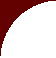Converting Liters to Pounds of MilkFARMLLC home

Milk: Liters to Pounds

HOW TO CONVERT MILK VOLUMES AND PRICES TO THOSE IN THE U.S.

Typical Dairy Cows in Malawi

# How to convert liters to pounds of milk:

(In the U.S., dairy farmers are paid per 100 lb of milk;  1 cwt = 100 lb)

Raw milk doesn't weigh the same as water because of the solids content of milk, so you can't do a straight conversion of mass to volume:

Milk weighs 8.6 lb/gallon (U.S. gallon)

There are 3.7854 liters/U.S. gallon

Therefore:    8.6 lb/ 3.7854 liters = 2.272 lb/liter of milk

Therefore:  100 lb/2.272 lb = 44.01 liters/cwt milk   (i.e., there are 44 liters/cwt milk)

------------------------------------------------------------------------------------------------------

If milk price is \$25/cwt in the U.S., this converts to a price of US\$25/44 = US\$.5680/liter

If milk price in \$20/cwt in the U.S., this converts to a price of US\$20/44 = US\$.4544/liter

If milk price is \$17/cwt in the U.S., this converts to a price of US\$17/44 = US\$.3862/liter

If milk price in \$15/cwt in the U.S., this converts to a price of US\$15/44 = US\$.3408/liter

If milk price in \$12/cwt in the U.S., this converts to a price of US\$12/44 = US\$.2726/liter

(i.e., divide US\$/cwt by 44 to get US\$/liter)

------------------------------------------------------------------------------------------------------

If milk price is \$.60/liter, this converts to a price of \$26.41/cwt in the U.S.

If milk price is \$.50/liter, this converts to a price of \$22.00/cwt in the U.S.

If milk price is \$.40/liter, this converts to a price of \$17.61/cwt in the U.S.

If milk price is \$.30/liter, this converts to a price of \$13.20/cwt in the U.S.

If milk price is \$.25/liter, this converts to a price of \$11.00/cwt in the U.S.

(i.e., multiply US\$/liter by 44 to get US\$/cwt)

------------------------------------------------------------------------------------------------------

5 liters of milk = 11.36 lb                        10 lb of milk = 4.40 liters

7.5 liters of milk = 17.04 lb                        15 lb of milk = 6.60 liters

10 liters of milk = 22.72 lb                         20 lb of milk =  8.80 liters

15 liters of milk = 34.08 lb                         30 lb of milk = 13.20 liters

20 liters of milk = 45.44 lb                         40 lb of milk = 17.60 liters

25 liters of milk = 56.80 lb                         50 lb of milk = 22.01 liters

30 liters of milk = 68.16 lb                         60 lb of milk = 26.40 liters